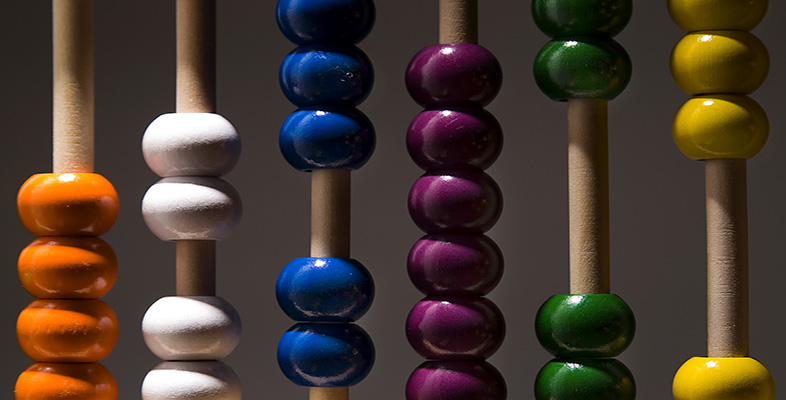Presenting information

Start this free course now. Just create an account and sign in. Enrol and complete the course for a free statement of participation or digital badge if available.

Free course

# 1.2.4 Rounding

It looks as if there was an extra 0.2 of a person in the last calculation – why was this? Probably because the figure of 85% that we used was not precise. In fact 809 people, as a percentage of 952, is very slightly less than 85%.

Values are often rounded, and using these rounded numbers in calculations can give answers like 809.2 people. Here we can round the answer down to 809 people, because we know it must be a whole number and it is more likely to be 809 than 810.

When we want to give a number as a whole number, a decimal that is greater than 0.5 is rounded up, and a decimal that is less than 0.5 is rounded down. A decimal that is exactly 0.5 is usually rounded up.

We can use the 809.2 people as an example to show how this is done. Because we are rounding to a whole number, we need to look at the decimal part, which is .2. This is less than 0.5, so we round down, giving us 809 people. However, if the number of people had been 809.7, we would round up to give 810.

Rounding is not only used to make whole numbers. Often rounding is used to make long decimal numbers shorter. For example, we could round 63.87 up to 63.9.

In fact, the figure of 85% (people using email) must itself have been rounded to give a whole-number percentage. This means that it could originally have been any percentage greater than 84.5% and less than 85.5%. The precise result of expressing 809 people as a percentage of 952 is a long number beginning 84.9789, and going on for many more decimal places. It makes sense to round this percentage up to 85% in this context. (But if greater precision were required, rounding to 85% might be unacceptable.) If we use the same method of calculation as before we can work out that this 85% could represent any number of people between 804 and 813.

## Activity 6 (self-assessment)

Assuming that 952 people had used the internet in the previous three months, use the percentages given in Table 1 to calculate the actual number of people who had used the internet for the following purposes:

(Round the resulting figures to the nearest whole number.)

1. General browsing

2. Selling goods and services

1. 65% of people used the internet for general browsing; this is 65% of 952 people. Entering '65 / 100×952 =' into the Windows calculator gives 618.8. This figure rounds up to 619 people.

2. 10% of people used the internet used for selling goods or services; this is 10% of 952 people. Entering '10 / 100×952 =' into the calculator gives 95.2. This figure is rounded down to 95.

3. 13% of people used the internet for playing or downloading games; this is 13% of 952 people or '13 / 100×952', which gives 123.76. The decimal part of this number is .76, which is bigger than 0.5, so we round up to 124.

T175_7